# Numerical study of nanofluid heat transfer SiO2 through a solar flat plate collector

Numerical study of nanofluid heat transfer SiO2 through a solar flat plate collector

Ammar Maouassi*Abdelhadi Baghidja Said Daoud Noureddine Zeraibi

Department of Mechanical Engineering, University of Constantine1, Constantine 25000, Algeria

Renewable Energy and Sustainable Development Laboratory (LERDD), University of Constantine1, Constantine 25000, Algeria

Department of Transportation of Hydrocarbons, University of Boumerdès, Boumerdès 35000, Algeria

Corresponding Author Email:
maouassi.ammar@umc.edu.dz
Page:
619-625
|
DOI:
https://doi.org/10.18280/ijht.350319
|
Accepted:
|
Published:
30 September 2017
| Citation

OPEN ACCESS

Abstract:

This paper illustrates how practical application of nanoparticles (SiO2) as working fluid to stimulate solar flat plate collector efficiency with heat transfer modification properties. A numerical study of nanofluids laminar forced convection, permanent and stationary (SiO2), is conducted in a solar flat plate collector. The effectiveness of these nanofluids are compared to conventional working fluid (water), wherein the dynamic and thermal properties are evaluated for four volume concentrations of nanoparticles (1%, 3%, 5% and 10%), and this done for Reynolds number from 25 to 900. Results from the application of those nonfluids are obtained versus average temperature; pressure drop coefficient and Nusselt number are discussed later in this paper. Finally, we concluded that heat transfer increases with increasing both nanoparticles concentration and Reynolds number.

Keywords:

solar energy, CFD, nanofluid, heat transfer, sio2 nanoparticles, solar flat plate collector

1. Introduction

Thermal solar energy has a major importance in the world because it’s clean, renewable and sustainable, it has several practical and industrial applications, including electrical production, energy systems heating and cooling; in which the principal elements in these systems are the solar collectors which convert solar radiation to thermal energy then transfer it towards the carrying fluid . The most known solar collectors used are the flat-plat collector. However, this type has relatively low energy efficiency and low outlet temperatures. Recently, several studies have been carried out on the flat plate solar collector to overcome these disadvantages [2, 3], in addition to these classical studies or methods, which are used to increase the thermal efficiency of this kind of solar collector, one of the latest effective method is to replace the conventional working fluid by other fluids, which having higher thermal properties.

The idea to improve thermo-physical properties of conventional fluids is adding solid particles with a high thermal characteristics and nano-size, inside basic fluid. This new generation of fluids named Nanofluids; this term was introduced first by Choi , and usually stills use to characterize this colloidal suspension type. After these initiative studies of the ARGONNE laboratory group, several studies have been carried out in this research area to understand the thermohydraulic behavior of this new heat transfer fluid generation [5-12]. Nanoparticles suspension within the conventional heat transfer fluid increases its thermal conductivity and consequently improves the heat transfer . Free convection heat transfer in a porous medium with nanofluid was examined by Sheikholeslami et al. [6, 7] they found that the improvement of the heat transfer coefficient is related to the increase of nanoparticles volume concentration.

In the same research field context, Sheikholeslami  carried out a 3D numerical study on forced convection heat transfer, it is found that the temperature gradient on the hot surface side increases with the increase of the nanoparticles volume fraction and both Reynolds and Darcy numbers. The magnetic field Influence on nanofluids hydrothermal behavior, Sheikholeslami used two different nanofluid types, Fe3O4-H2O nanofluid [9, 11] and CuO-H2O nanofluid [5, 6, 12]. These studies demonstrate when Nusselt number increases, it has a direct relation with Rayleigh number and nanoparticles volume concentration; whereas inverse relation with Hartmann number when decreasing. Despite the fact that there is no commercial solar collector available that uses these nanofluids, there are experimental studies that confirm its effectiveness by adding nanoparticle to improve the thermal efficiency, Natarajan et al.  found that if the nanofluids are used as working fluids, the energy efficiency of solar water heaters increases significantly compared to conventional fluids. On the other hand, the dispersion of the nanoparticles gives an important increase in the heat transfer coefficient [14-19]. Hence, they found that it is possible to reduce the exchange surface and improve the efficiency of these devices, which confirms the results of . Nasrin et al.  carried out a numerical study of forced convection heat transfer through a flat plate solar collector, the geometry is tested for 2D case, then 3D, where water and Cu-H2O nanofluid are used as a working fluid, the results obtained show that 3D simulation is more efficient than 2D, and the increase of nanoparticles volume concentration gives an improvement in heat transfer rate reach 17% and about 8% for thermal efficiency of solar collector.

In this paper, we present the study of heat transfer of nanofluid (SiO2) flows through a solar flat plate collector. In the first part, we describe the problem and present the boundary conditions. After grid independence study, then we validated the results by comparing them with previous literature reports.

The key part of this work is to involve the simulation with the interpretation of numerical results obtained for this case, where the effect of nanoparticles is shown for various volume concentration and Renolds number, and studied systematically. At the end, this paper is footed by conclusion which summarizes the main results obtained.

2. Mathematical Formulation
3. Numerical Method and Code Validation

To conduct numerical simulation, the computational domain was meshed with control volumes built around each grid using GAMBIT (version 2.4.6), which is the pre-processor for FLUENT (version 6.3). Numerical simulation was carried out using steady state implicit pressure based solver, which is an in-built in the commercially available software FLUENT (version 6.3). Governing partial differential equations, for mass and momentum, are solved for the steady incompressible flow. The velocity-pressure coupling is affected through SIMPLE algorithm developed by Patankar . Second order upwind schemes were chosen for the solution schemes laminar flow condition was used.

3.1 Meshing

The computational domain was meshed and done by using appropriate grid cells, with suitable size. Three dimensional computational domains are built and used, as shown in Fig. 1. To solve the previous system of governing equations (1-3), the computational domain was meshed with the ﬁnite control volume method  which has been successfully used by several authors [29, 30]. To compute the combined convection-and-diffusion ﬂuxes of heat the upwind method are used, where the upwind scheme of the second order has been used throughout for computing heat and momentum fluxes.

## 1.pngFigure 1. Mesh of the computational domain

## 2.png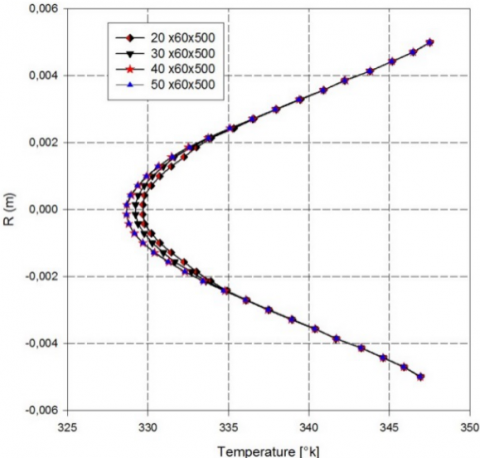Figure 2. Mesh validation curves for the outlet temperature evolution

Several non-uniform grids have been thoroughly tested to ensure the accuracy and the consistency of numerical results, which has shown that the 40 x 60x 500 non-uniform grid (32, 24 and 155 nodes, respectively, along the radial, tangential and axial directions) appears satisfactory for the absorber tube (fig. 2); with refining mesh near the tube wall and the entrance region.

3.2 Code validation

Figure 3 represent the temperature distribution on the absorber plate for different values of inlet velocity (V= 0.001, V= 0.005, V= 0.01 and V= 0.05 m/s). The axial temperatures evolution of the working fluid along the absorber tube are represented in Fig. 4; with different axial flow velocities VZ ranging from 0.0001 to 0.05 m/s, in the same boundary conditions used by Karanth et al. .

## 3.pngFigure 3. Temperature distribution on the absorber for (a) V= 0.001m/s, (b) V=0.005m/s, (c) V=0.01m/s, (b) V=0.05m/s

## 4.png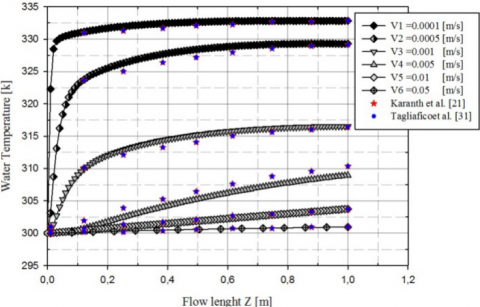Figure 4. Validation of temperature contour of absorber plate Comparison of fluid temperature distribution between this study and these of Karanth et al.  & Tagliafico et al. 

The numerical results obtained by Karanth et al. , provide an excellent test tool to validate our calculation code; by comparing the obtained numerical results with those published by Karanth et al.  and Tagliafico et al.  literatures, we notice a very good matching between our results and those of the authors; hence our simulation is successfully validated.

4. Results and Discussion

We study the nanofluids heat transfer flow inside an absorbent tube, where he heated by the absorbent plaque. We consider the flow regime as laminar, and varying the number of Reynolds between 25 and 900, for the different nanoparticles concentrations (1%, 3%, 5%, and 10%). Then we plot the results, pressure drop coefficient and Nusselt number.

4.1 Pressure drop coefficient

Figures 5.a and 5.b, represent the variation of skin friction coefficient versus volume concentration "f" and Reynolds number "Re". We note a slight increase in skin friction coefficient (λ) when the Reynolds number is low (Re ≤ 100) as showing in Fig. 5.a, this slight increase in λ is due to the presence of the nanoparticles and lower fluid flow velocities, but when the Reynolds number exceeds 100 this problem doesn’t occur.

## 5a.png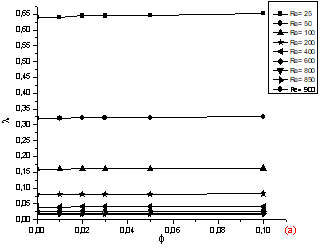（a）

## 5b.png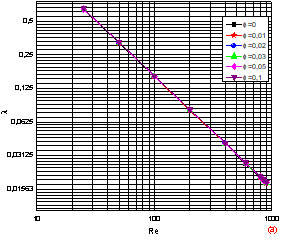（b）

Figure 5. Skin friction coefficient versus: (a) volume fraction of SiO2 nanoparticles and (b) Reynolds numbers

Fig. 5.b, it will be noted that for the same Reynolds number, and despite the variation in the nanoparticles concentration volume; the λ value is almost identical, this confirm and supports the previous result in Fig. 5.a. These results, therefore, reflect the insignificant influence of the nanoparticles volume concentration on the skin friction coefficient λ.

4.2 Nusselt number

## 6a.png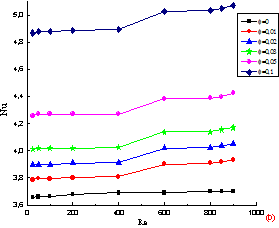（a）

## 6b.png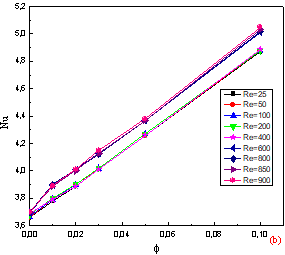Figure 6. Heat transfer coefficient versus: (a) Reynolds number and (b) different concentrations of SiO2 nanoparticles

## 7.png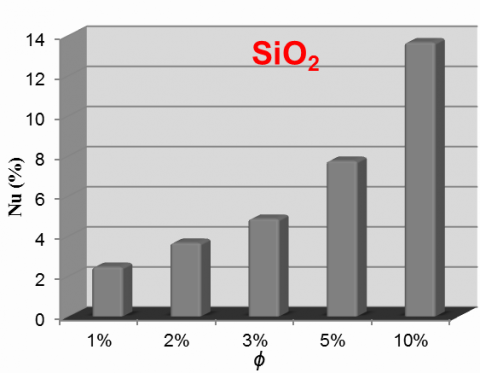Figure 7. The improvement rate of heat transfer coefficient

Figures 6.a and 6.b, represents the Nusselt number "Nu" evolution as a function of Reynolds number and the volume fraction "f".

We note, a significant increase of Nusselt number with the increase in nanoparticle volume concentration for all its value as showing in figure 6.a, so in relation with pure water, whereas, a slight increase versus Reynolds number as showing in figure 6.b. Each increase in the volume concentration is accompanied with a significant increase in the Nusselt number, consequently the heat transfer intense through the working fluid.

In the Figure 7, represent the comparison in term of rate improvement of heat transfer coefficient for various volume concentrations of nanoparticles.

We notice then, a considerable increase of the improvement rate compared to pure water which can reach an average value of 13.7 % for f= 10 %.

5. Conclusions

In this paper, the flow and heat transfer characteristics of SiO2-water nanofluid for arrange of the Reynolds number (25to 900) with a wide range of volume concentration (0 to 10%) are studied numerically.

We can confirm from analyze of thermal-hydraulic properties of nanofluids, on one hand, the presence of nanoparticles in the base fluid (pure water) increases significantly Nusselt number, that is also growing with the increase of volume fraction, the increase in heat coefficient results improve the heat transfer through the energy systems compared to the base fluid (pure water) case. On the other hand, the gain in heat is accompanied by a slight increase in skin friction coefficient for the low Reynolds numbers due to the presence of the nanoparticles and lower flow velocities, but when the Reynolds number exceeds 100 this problem doesn’t occur.

The results show that, Nusselt number and heat transfer coefficient, of nanofluid are strongly dependent on nanoparticle and increase by increasing of the volume concentration of nanoparticles, and the insignificant influence of the nanoparticles volume concentration on the skin friction coefficient.

Nomenclature
 Latins Symbols Cp Speciﬁc heat h Heat transfer coefficient k Thermal conductivity T Temperature L Length of the absorber tube D Diameter of the absorber tube Nu Nusselt number Re Reynolds number u axial velocity R tube radius q″ heat flux Greek symbols ϕ Volume concentration λ skin friction coefficient µ Viscosity ρ Density Subscripts s Solid f Fluid nf Nanofluid m mean value w wall sf surface
References

 Rovense F., Amelio M., Ferraro V., Scornaienchi N.M. (2016). Analysis of a concentrating solar power tower operating with a closed joule Brayton cycle and thermal storage, International Journal of Heat and Technology, Vol. 34, No. 3, pp. 485-490. DOI: 10.18280/IJHT.340319

 Nasrin R., Alim M.A. (2015). Thermal performance of nanofluid filled solar flat plate collector, International Journal of Heat and Technology, Vol. 33, No. 2, pp. 17-24. DOI: 10.18280/IJHT.330203

 Zhuang C., Fu B., Huang G., Zhang H. (2016). Optimization of the structure of a solar air heater fitted with V-shaped perforated baffles, International Journal of Heat and Technology, Vol. 34, No. 4, pp. 604-610. DOI: 10.18280/IJHT.340408

 Choi S.U.S., Eastman J.A. (1995). Enhancing thermal conductivity of fluids with nanoparticles, ASME Int Mech Eng Congr Expo, San Francisco, USA, Vol. 66, pp. 99-105.

 Sheikholeslami M. (2014). Effect of uniform suction on nanofluid flow and heat transfer over a cylinder, J Braz. Soc. Mech. Sci. Eng, Vol. 37, pp. 1623-1633. DOI: 10.1007/S40430-014-0242-Z

 Sheikholeslami M. (2017). Influence of magnetic field on nanofluid free convection in an open porous cavity by means of lattice Boltzmann Method, Journal of Molecular Liquids, Vol. 234, pp. 364-374. DOI: 10.1016/J.MOLLIQ.2017.03.104

 Sheikholeslami M. (2017). CuO-water nanofluid free convection in a porous cavity considering Darcy law, Eur. Phys. J. Plus, Vol. 132, pp. 1-11. DOI: 10.1140/EPJP/I2017-11330-3

 Sheikholeslami M. (2017). Magnetohydrodynamic nanofluid forced convection in a porous lid driven cubic cavity using Lattice Boltzmann Method, Journal of Molecular Liquids, Vol. 231, pp. 555-565. DOI: 10.1016/J.MOLLIQ.2017.02.020

 Kandelousi M.S. (2014). Effect of spatially variable magnetic field on ferrofluid flow and heat transfer considering constant heat flux boundary condition, Eur. Phys. J. Plus, Vol. 129, pp. 1-12. DOI: 10.1140/EPJP/I2014-14248-2

 Sheikholeslami M. (2017). Magnetic field influence on nanofluid thermal radiation in a cavity with tilted elliptic inner cylinder, Journal of Molecular Liquids, Vol. 229, pp. 137-147. DOI: 10.1016/J.MOLLIQ.2016.12.024

 Sheikholeslami M. (2017). Numerical simulation of magnetic nanofluid natural convection in porous media, Physics Letters A, Vol. 381, pp. 494-503. DOI: 10.1016/J.PHYSLETA.2016.11.042

 Sheikholeslami M. (2017). Influence of Lorentz forces on nanofluid flow in a porous cylinder considering Darcy model, Journal of Molecular Liquids, Vol. 225, pp. 903-912. DOI: 10.1016/J.MOLLIQ.2016.11.022

 Natarajan E., Sathish R. (2009). Role of nanofluids in solar water heater, Int J. Adv Manuf Technol, Vol. 45, pp. 1-5. DOI: 10.1007/S00170-008-1876-8

 Sokhansefat T., Kasaeian A.B., Kowsary F. (2014). Heat transfer enhancement in parabolic trough collector tube using Al2O3/synthetic oil nanofluid, J. Renewable and Sustainable Energy Reviews, Vol. 33, pp. 636-644. DOI: 10.1016/J.RSER.2014.02.028

 Otanicar T.P., Phelan P.E., Prasher R.S., Rosengarten G., Taylor R.A. (2010). Nanofluid-based direct absorption solar collector, J. Renewable and Sustainable Energy, Vol. 2, p. 13. DOI: 10.1063/1.3429737

 Faizal M., Saidur R., Mekhilef S., Alim M.A. (2013). Energy, economic and environmental analysis of metal oxides nanofluid for flat-plate solar collector, J. Energy Conversion and Management, Vol. 76, pp. 162-168. DOI: 10.1016/J.ENCONMAN.2013.07.038

 Said Z., Sajid M.H., Alim M.A., Saidur R., Rahim N.A. (2013). Experimental investigation of the thermophysical properties of AL2O3-nanofluid and its effect on a flat plate solar collector, Int Commu in Heat and Mass Transfer, Vol. 48, pp. 99-107. DOI: 10.1016/J.ICHEATMASSTRANSFER.2013.09.005

 He Q., Zeng S., Wang S. (2015). Experimental investigation on the efficiency of flat-plate solar collectors with nanofluids, J. Applied Thermal Engineering, Vol. 88, pp. 165-171. DOI: 10.1016/J.APPLTHERMALENG.2014.09.053

 Menbari A., Alemrajabi A.A., Rezaei A. (2016). Heat transfer analysis and the effect of CuO/Water nanofluid on direct absorption concentrating solar collector, J. Applied Thermal Engineering, Vol. 104, pp. 176-183. DOI: 10.1016/J.APPLTHERMALENG.2016.05.064

 Nasrin R., Alim M.A., Ahmed S.R. (2016). Comparative study between 2D and 3D modeling of nanofluid filled flat plate solar collector, International Journal of Heat and Technology, Vol. 34, No. 3, pp. 604-610. DOI: 10.18280/IJHT.340326

 Karanth K., Manjunath M., Sharma N. (2011). Numerical simulation of a solar ﬂat plate collector using discrete transfer radiation model (DTRM) – a CFD approach, In: Proceedings of the world congress on engineering, London, pp. 2355-2360.

 Xuan Y., Roetzel W. (2000). Conception for heat transfer correlation of nanofluids, Int J. Heat and Mass Transfer, Vol. 43, pp. 3701-3707. DOI: 10.1016/S0017-9310(99)00369-5

 Pak B.C., Cho Y.I. (1998). Hydrodynamic and heat transfer study of dispersed fluids with submicron metallic oxide particles, J. Experimental Heat Transfer, Vol. 11, pp. 151-170. DOI: 10.1080/08916159808946559

 Zhang X., Gu H., Fujii M. (2007). Effective thermal conductivity and thermal diffusivity of nanoﬂuids containing spherical and cylindrical nanoparticles, J. Experimental Thermal and Fluid Science, Vol. 31, pp. 593-599. DOI: 10.1016/j.expthermflusci.2006.06.009

 Brinkman H.C. (1952). The viscosity of concentrated suspensions and solution, J. Chemistry Physics, Vol. 20, pp. 571-581. DOI: 10.1063/1.1700493

 Maxwell J.C. (1892). A Treatise on Electricity and Magnetism, 3rd ed., Vol. 2, Clarendon Press, Oxford, 1904, pp. 430-441.

 Patankar V.S. (1980). Numerical Heat Transfer and Fluid Flow, 1st ed., Hemisphere Publishing Corporation, New York, pp. 113-125.

 Versteeg H., Malalasekera W. (1996). An Introduction to Computational Fluid Dynamics: The Finite Volume Method, 2nd ed., Longman Group Lt, England, pp. 85-133.

 Beyers J.H.M., Harms T.M., Kröger D.G. (2001). A finite volume analysis of turbulent convective heat transfer for accelerating radial flows, J. Numerical Heat Transfer, Vol. 40, pp. 117-138. DOI: 10.1080/104077801750468453

 Maïga S.E.B., Nguyen C.T., Galanis N., Roy G. (2004). Heat transfer behaviors of nanofluids in uniformly heated tube, Superlatives and Microstructures, Vol. 35, pp. 543-557. DOI: 10.1016/J.SPMI.2003.09.012

 Tagliafico I.A., Scarpa F., Rosa M.D. (2014). Dynamic thermal models and CFD analysis for flat-plate thermal solar collectors – a review, Renew and Sust Energy Rev, Vol. 2, No. 30, pp. 526-537. DOI: 10.1016/J.RSER.2013.10.023